# Fejér summation method

(diff) ← Older revision | Latest revision (diff) | Newer revision → (diff)

A summation method of arithmetical averages (cf. Arithmetical averages, summation method of), applied to the summation of Fourier series. It was first applied by L. Fejér .

The Fourier series(1)

of a function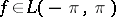is summable by the Fejér summation method to a functionifwhere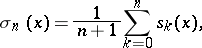(2)

and the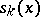are the partial sums of (1).

Ifis a point of continuity ofor a discontinuity of the first kind, then its Fourier series at that point is Fejér summable toor to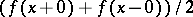, respectively. Ifis continuous on some interval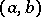, then its Fourier series is uniformly Fejér summable on every segment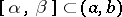; and ifis continuous everywhere, then the series is summable touniformly on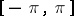(Fejér's theorem).

This result was strengthened by H. Lebesgue , who proved that for every summable function, its Fourier series is almost-everywhere Fejér summable to.

The function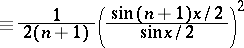is called the Fejér kernel. It can be used to express the Fejér means (2) ofin the form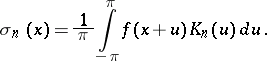How to Cite This Entry:
Fejér summation method. Encyclopedia of Mathematics. URL: http://encyclopediaofmath.org/index.php?title=Fej%C3%A9r_summation_method&oldid=18036
This article was adapted from an original article by I.I. Volkov (originator), which appeared in Encyclopedia of Mathematics - ISBN 1402006098. See original article